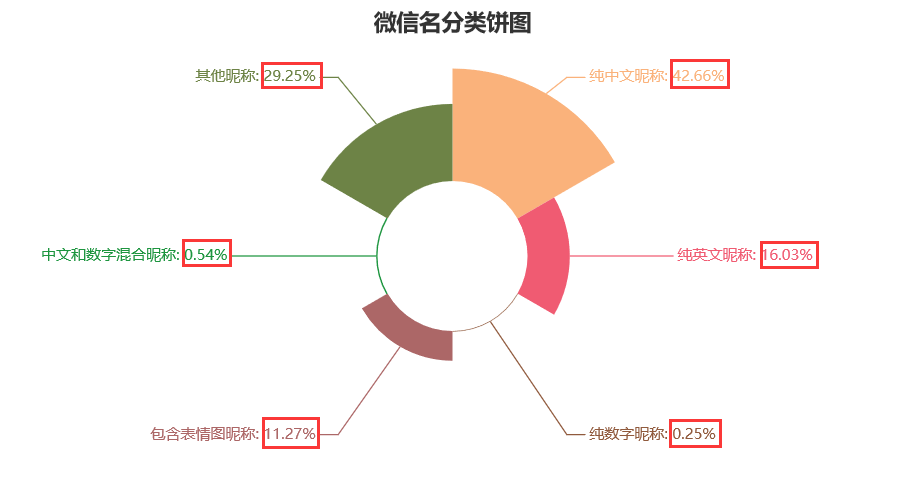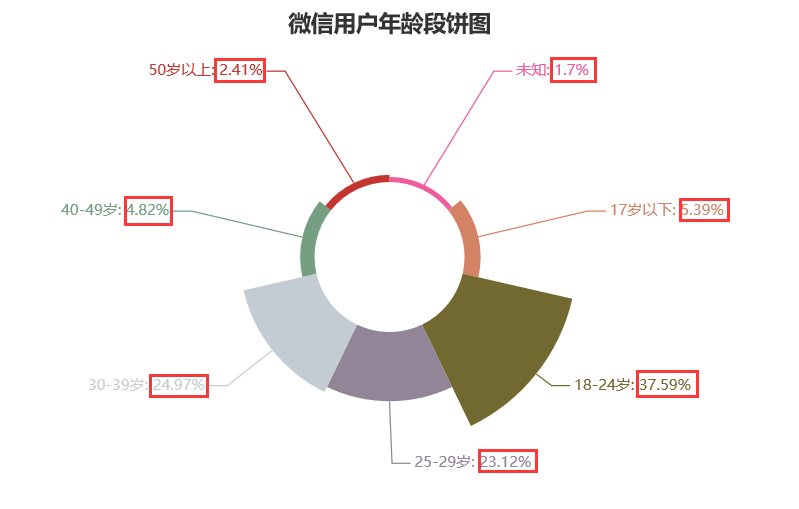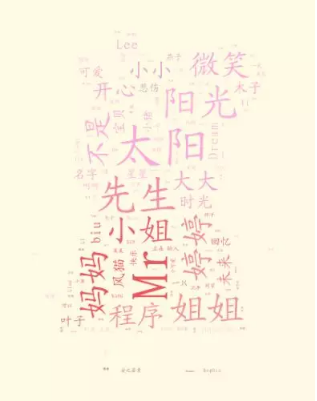Java帮帮-微信公众号Java帮帮-微信公众号将分享做到极致 职业司微信公众号职业司微信公众号实时动态通知 程序员生活志-公众号程序员生活志-公众号程序员生活学习圈，互联网八卦黑料

 编程资讯
 Java
 程序员段子
 程序人生
 秒杀专栏
 名企资讯
 运维监控
 人事面试
 技术面试
 大禹编程
 UI
 SpringBoot
 SpringCloud(F版)
 SpringCloud(D版)
 spring
 Docker
 Dubbo
 IntelliJ IDEA
 Linux
 Nginx
 Tomcat
 Quartz
 微信小程序
 阿里云
 Python
 Java案例
 小程序源码
 Java源码
 手机资讯
 Sql Server
 MySQL
 MongoDB
 前端开发
 node.js
 CSS
 移动端
 jQuery
 Sublime Test
 JavaScript
 HTML
 Vue.js
 HTTPS
 分布式事物
 Redis
 JSON
 DevOps
 情感人生
 黑科技
 Oracle
 Git
 微服务
 大数据
 职业生涯
 人工智能
 Keras教程
 RocketMQ
 PyTorch教程
 Spring Security

# Python分析 | 从00-90后的微信昵称

25

### 二、Let's get it

#### 1.基本信息获取

1.访问`英文取名`的用户基本信接口，获取`英文取名`用户微信名（`NickName`）、访问次数（`Count`）、总数据集（`ResponseData`），并将微信名存入文件。

``# 获取所有用户数量和相关信息def get_json():    # 获取入口    search_url = '英文取名用户接口，欢迎扫码使用英文取名，生成一个最适合你的英文名'    # 发送http请求,获取请求页面    search_response =requests.get(search_url)    # 设置编码    search_response.encoding ='UTF-8'    # 将页面转变成json代码格式    search_json =search_response.json()    # 获取我们需要的数据,是列表格式    our_data =search_json['ResponseData']    list_len = len(our_data)    print('总用户数有：' + str(list_len))    user_visit_numbers = 0    data_research = 0    NickName = []    for x in our_data:        user_numbers = x['Count'] +user_visit_numbers        if x['NickName'] == '':            data_research += 1       NickName.append(x['NickName'])    print("微信名获取失败量："+str(data_research))    print(NickName)    name = ['微信名称']    file_test =pd.DataFrame(columns=name, data=NickName)    file_test.to_csv(r'I:/data.csv',encoding='utf-8',index=False)    print('总访问量：' + str(user_visit_numbers))``

##### 2.读取所有微信名，数据分类
###### (1)读取微信名
``# 读取文件，取出微信名def get_name():    NickName = []    with open('I:/data.csv','r',encoding='utf8') as file :        i = 0        for line in file:            if i == 0:   # 去除表头                i = 1                continue            line = line.strip()    # 去除换行符            NickName.append(line)    return NickName``
###### (2)数据分为以下六大类

 全中文昵称 ch_name list 全英文昵称 en_name list 中文和数字混合昵称 ch_di_name list 包含图片表情昵称 img_name list 其他昵称 other_name list

``# ch ：Chinesech_name_number = 0ch_name = []# en ：Englishen_name_number = 0en_name = []# di : digtaldi_name_number = 0di_name = []# img : imageimg_name_number = 0img_name = []# ch_di : Chinese and digtalch_di_name = []# other : otheroth_name_number = 0oth_name = []``
###### (3)数据分类判断
``# 昵称全中文判断def is_all_ch(keyword):    for c in keyword:        # 包含常见中文字符        if not ('\u4e00' <= c <= '\u9fa5'):            return False    return True# 昵称全英文判断def is_all_en(keyword):    # 不能全部为空格或者首位为空格    if all(ord(c) == 32 for c in keyword) or keyword == ' ':        return False    # 允许空格和英文并存(例如：Xist A)    if not all(65 < ord(c) < 128 or ord(c) == 32 for c in keyword):        return False    return True# 昵称全数字判断def is_all_di(keyword):    for uchar in keyword:        if not (uchar >= '\u0030' and uchar <= u'\u0039'):            return False    return True# 昵称包含表情图判断def have_img(keyword):    # 下面是大部分图片的一个unicode编码集    # 详情查看：https://en.wikipedia.org/wiki/Emoji    img_re = re.compile(u'['                      u'\U0001F300-\U0001F64F'                      u'\U0001F680-\U0001F6FF'                      u'\u2600-\u2B55]+',                      re.UNICODE)    if img_re.findall(keyword) :        return True    return False# 中文+数字昵称判断def is_ch_di(keyword):    for c in keyword:        if not ('\u4e00' <= c <= '\u9fa5') and not (c >= '\u0030' and c <= u'\u0039'):            return False    return True``
###### (4)数据归类计算各类数量
``list_name = get_name()print("总共有："+str(len(list_name))+"个微信名")for i in range(len(list_name)):    result = classification_name(list_name[i])    if result == 'ch':  # 中文        ch_name_number +=1        ch_name.append(list_name[i])    if result == 'en':  # 英文        en_name_number +=1        en_name.append(list_name[i])    if result == 'di':  # 数字        di_name_number +=1        di_name.append(list_name[i])    if result == 'img': # 含表情        img_name_number +=1        img_name.append(list_name[i])    if result == 'ch_di': # 中文和数字        ch_di_name_number +=1        ch_di_name.append(list_name[i])    if result == 'other': # 其他        oth_name_number +=1        oth_name.append(list_name[i])print("纯中文昵称个数："+ str(ch_name_number))# print(ch_name)print("纯英文昵称个数："+ str(en_name_number))#print(en_name)print("纯数字昵称个数："+ str(di_name_number))# print(di_name)print("包含表情图昵称个数："+ str(img_name_number))# print(img_name)print("中文和数字混合昵称个数："+ str(ch_di_name_number))print(ch_di_name)print("其他昵称个数："+ str(oth_name_number))# print(oth_name)``

##### 3.获取用户画(只获取用户年龄段)

3.访问英文取名用户画像接口，获取近30天活跃用户和新用户的年龄段

``# 获取用户年龄段def get_data():    # 获取token,并处理    t = get_token().strip('"')    # 然后将处理后的token值和其他参数作为post方式的参数值，调用用户画像api    post_user_api = " https://api.weixin.qq.com/datacube/getweanalysisappiduserportrait?access_token="    post_user_url = post_user_api + t    # 访问获取概况数据 (近一个月的数据情况)    data = json.dumps({    "begin_date" : "2018-07-21",    "end_date" : "2018-08-19"})    # 获取信息    user_portrait_data = get_info(post_user_url, data)    # 时间段    ref_date = user_portrait_data['ref_date']    # 新用户    visit_uv_new = user_portrait_data['visit_uv_new']     活跃用户    visit_uv = user_portrait_data['visit_uv']    # 年龄段    print(ref_date )    print((visit_uv_new['ages']))    print((visit_uv['ages']))``

``# id ： 为年龄段序号  name ：年龄段名称    value : 该年龄段人数20180721-20180819[{'id': 0, 'name': '未知', 'value': 6}, {'id': 1, 'name': '17岁以下', 'value': 18}, {'id': 2, 'name': '18-24岁', 'value': 118}, {'id': 3, 'name': '25-29岁', 'value': 75}, {'id': 4, 'name': '30-39岁', 'value': 81}, {'id': 5, 'name': '40-49岁', 'value': 14}, {'id': 6, 'name': '50岁以上', 'value': 7}][{'id': 0, 'name': '未知', 'value': 6}, {'id': 1, 'name': '17岁以下', 'value': 20}, {'id': 2, 'name': '18-24岁', 'value': 147}, {'id': 3, 'name': '25-29岁', 'value': 88}, {'id': 4, 'name': '30-39岁', 'value': 95}, {'id': 5, 'name': '40-49岁', 'value': 20}, {'id': 6, 'name': '50岁以上', 'value': 10}]``

### 三、来点有趣的，数据清洗、分析

##### 1.微信名称类型数据可视化分析

``# 1.微信名分类：玫瑰饼图from pyecharts import Pie# 数据获取自上面代码attr = ["纯中文昵称", "纯英文昵称", "纯数字昵称", "包含表情图昵称", "中文和数字混合昵称", "其他昵称"]v1 = [1514, 569, 9, 400, 19, 1038]pie = Pie("微信名分类饼图", title_pos='center', width=900)pie.add(    "占比",    attr,    v1,    center=[50, 50],    is_random=True,    radius=[30, 75],    rosetype="area",    is_legend_show=False,    is_label_show=True,)pie.render("render_01.html")``#### 2.微信用户年龄段可视化分析

``# 2.用户年龄段：玫瑰饼图from pyecharts import Pie# 数据获取自上面代码attr = ["未知", "17岁以下", "18-24岁", "25-29岁", "30-39岁", "40-49岁","50岁以上"]v1 = [12, 38, 265, 163, 176, 34,17]pie = Pie("微信用户年龄段饼图", title_pos='center', width=900)pie.add(    "占比",    attr,    v1,    center=[50, 50],    is_random=True,    radius=[30, 75],    rosetype="area",    is_legend_show=False,    is_label_show=True,)pie.render("render_02.html")``#### 3.词云分析微信名称哪些词语、表情包更受欢迎

(1)继续使用`pyecharts`生成词云图

``# 清洗数据，生成词云图def split_word(test_str):    test_str = re.sub('[,，。. \r\n]', '', test_str)    # jieba 词语    segment = jieba.lcut(test_str)    words_df = pd.DataFrame({'segment': segment})    # quoting=3 表示stopwords.txt里的内容全部不引用    stopwords = pd.read_csv(r"H:\PyCoding\ Analysis_wx_name\stopwords.txt", index_col=False, quoting=3, sep="\t", names=['stopword'], encoding='utf-8')    words_df = words_df[~words_df.segment.isin(stopwords.stopword)]    words_stat = words_df.groupby(by=['segment'])['segment'].agg({"计数": numpy.size})    words_stat = words_stat.reset_index().sort_values(by=["计数"], ascending=False)    test = words_stat.head(200).values    codes = [test[i] for i in range(0,len(test))]    counts = [test[i] for i in range(0,len(test))]    wordcloud = WordCloud(width=1300, height=620)    wordcloud.add("微信昵称", codes, counts, word_size_range=[20, 100])    wordcloud.render('render_03.html')``(2)使用`wordcloud`+`matplotlib`生成高级一点的词云图

``# 下下期好好讲一下matplotlib绘图可视化，挺有意思的# 调用get_name函数获取全部微信名text = get_name()# 调用jiebaclearText函数，清洗数据(该函数和上面切词思想一样)text1=jiebaclearText(text)#产生词云图bg = plt.imread(r"G:\small_pig.jpg")#生成词云wc=WordCloud(    background_color="wathet", #设置背景为白色，默认为黑色    mask=bg,      # 设置词云内容范围(除指定图片白色区域的其他区域都将覆盖词云内容)    margin=10,               #设置图片的边缘    max_font_size=70,   #显示的最大的字体大小    random_state=20,    #为每个单词返回一个PIL颜色    font_path='G:\simkai.ttf'   #中文处理，用系统自带的字体    # 可以在这里下载这个字体：http://www.font5.com.cn/font_download.php?id=534&part=1245067666    ).generate(text1)#为图片设置字体my_font=fm.FontProperties(fname='G:\simkai.ttf')# 图片背景bg_color = ImageColorGenerator(bg)# 开始画图plt.imshow(wc.recolor(color_func=bg_color))# 为云图去掉坐标轴plt.axis("off")# 保存云图wc.to_file("render_04.png")``### 四、通过昵称进行情绪分析(大胆猜想)

##### 01 | 微信昵称为全中文##### 02 | 微信昵称为全英文Java帮帮学习群生态

Java帮帮学习群生态

 Java学习群Java学习群与大牛一起交流 大数据学习群大数据学习群在数据中成长 九点编程学习群九点编程学习群深夜九点学编程 python学习群python学习群人工智能,爬虫
Java帮帮生态承诺

Java帮帮生态承诺